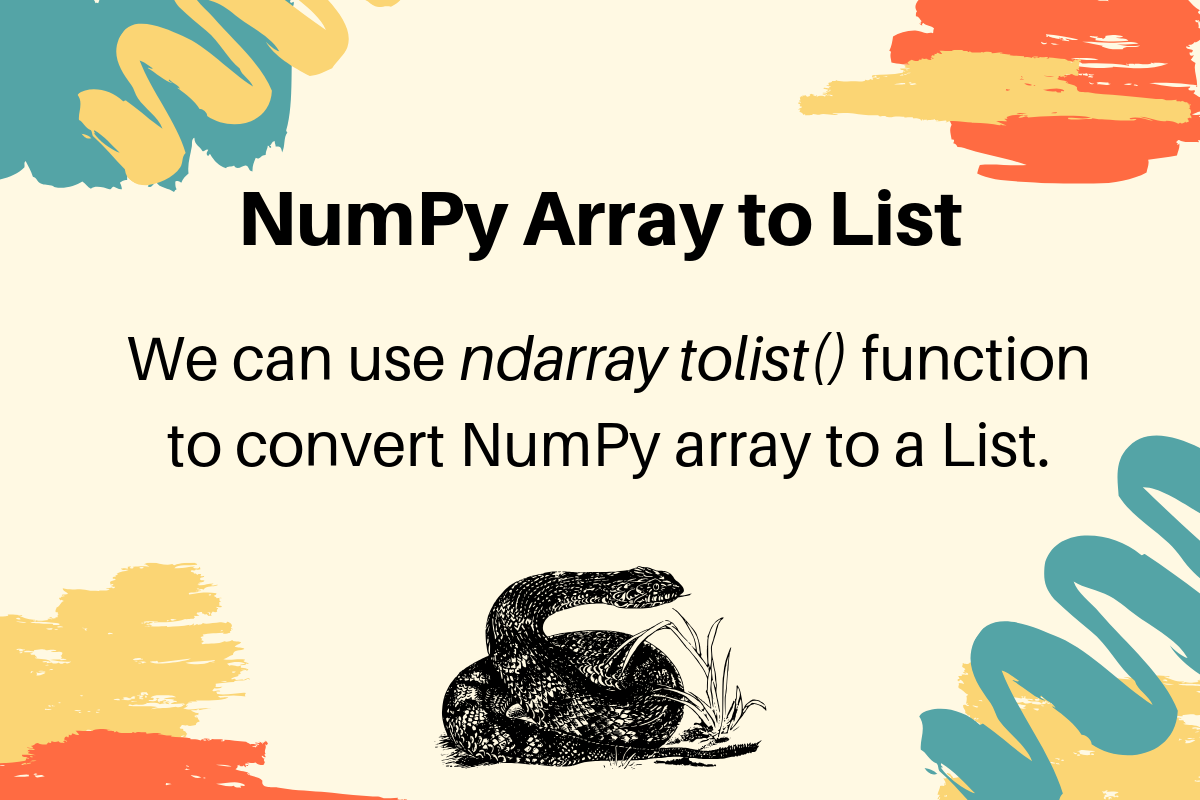# How To Convert a NumPy Array to List in Python

Published on August 3, 2022 · Updated on September 23, 2022By Pankaj### Introduction

With NumPy, `np.array` objects can be converted to a list with the `tolist()` function. The `tolist()` function doesn’t accept any arguments. If the array is one-dimensional, a list with the array elements is returned. For a multi-dimensional array, a nested list is returned.

## Prerequisites

In order to complete this tutorial, you will need:

This tutorial was tested with Python 3.9.6 and NumPy 1.23.3.

## Converting one-dimensional NumPy Array to List

Let’s construct a one-dimensional array of `[1, 2, 3]`:

``````import numpy as np

# 1d array to list
arr_1 = np.array([1, 2, 3])

print(f'NumPy Array:\n{arr_1}')
``````

This code will output:

``````NumPy Array:
[1 2 3]
``````

Now, let’s use `tolist()`:

``````import numpy as np

# 1d array to list
arr_1 = np.array([1, 2, 3])

print(f'NumPy Array:\n{arr_1}')

list_1 = arr_1.tolist()

print(f'List: {list_1}')
``````

This new code will output:

``````List: [1, 2, 3]
``````

The array has been converted from `numpy` scalars to Python scalars.

## Converting multi-dimensional NumPy Array to List

Let’s construct a multi-dimensional array of `[ [1, 2, 3], [4, 5, 6] ]`:

``````import numpy as np

# 2d array to list
arr_2 = np.array([[1, 2, 3], [4, 5, 6]])

print(f'NumPy Array:\n{arr_2}')
``````

This code will output:

``````NumPy Array:
[[1 2 3]
[4 5 6]]
``````

Now, let’s use `tolist()`:

``````import numpy as np

# 2d array to list
arr_2 = np.array([[1, 2, 3], [4, 5, 6]])

print(f'NumPy Array:\n{arr_2}')

list_2 = arr_2.tolist()

print(f'List: {list_2}')
``````

This new code will output:

``````List: [[1, 2, 3], [4, 5, 6]]
``````

The array has been converted from `numpy` scalars to Python scalars.

## Conclusion

In this article, you learned how to use `tolist()` to convert `np.array` objects to lists. It is applicable to one-dimensional and multi-dimensional arrays.

References

Thanks for learning with the DigitalOcean Community. Check out our offerings for compute, storage, networking, and managed databases.Pankaj

author

#### Still looking for an answer?

Ask a questionSearch for more help

JournalDev
DigitalOcean EmployeeAugust 7, 2020

thanks for your help man , great buddy . actualluy i was working in opencv2 and the value was in array and i cant turn it until i found that it was a numpy array . hahahahahah

- akshit

JournalDev
DigitalOcean EmployeeJuly 22, 2020

Thank you for this i can convert ndarray to list obj

- arnaldo

Click below to sign up and get \$200 of credit to try our products over 60 days!

### Popular Topics##### Hollie's Hub for Good

Working on improving health and education, reducing inequality, and spurring economic growth? We’d like to help.##### Become a contributor

You get paid; we donate to tech nonprofits.

##### Welcome to the developer cloud

DigitalOcean makes it simple to launch in the cloud and scale up as you grow – whether you’re running one virtual machine or ten thousand.© 2023 DigitalOcean, LLC.